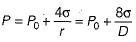Courses

# Test: Properties of Fluids - 3

## 20 Questions MCQ Test Topicwise Question Bank for Mechanical Engineering | Test: Properties of Fluids - 3

Description
This mock test of Test: Properties of Fluids - 3 for Mechanical Engineering helps you for every Mechanical Engineering entrance exam. This contains 20 Multiple Choice Questions for Mechanical Engineering Test: Properties of Fluids - 3 (mcq) to study with solutions a complete question bank. The solved questions answers in this Test: Properties of Fluids - 3 quiz give you a good mix of easy questions and tough questions. Mechanical Engineering students definitely take this Test: Properties of Fluids - 3 exercise for a better result in the exam. You can find other Test: Properties of Fluids - 3 extra questions, long questions & short questions for Mechanical Engineering on EduRev as well by searching above.
QUESTION: 1

### If angle of contact of a drop of liquid is acute than

Solution:

When adhesion force is more than cohesion force then fluid will wet the surface and angle of contact between fluid and surface will be less than 90° (acute).

QUESTION: 2

### What is unit of dynamic viscosity of a fluid termed as ’Poise’ equivalent to

Solution:

μ ⇒ Pa.s
1 poise = Dyne-sec/cm2

QUESTION: 3

### The mercury is known as non-wetting liquid because it does not wet the surface, The mercury posses this property due to

Solution:

Note that force between like molecules is known as cohesive force, and force between unlike molecules is known as adhesive force. Due to predominent cohesive force mercury posses non-wetting characteristic.

QUESTION: 4

Which one of the following is an example of Bingham plastic?

Solution:

Since some initial force is required to have the flow of tooth paste hence it is example of bingham plastic.

QUESTION: 5

Which one of the following is a typical example of non-newtonian fluid of Pseudo plastic variety?

Solution:

Milk, Blood ..........Pseudo plastic

QUESTION: 6

Match List-I (Physical properties of fluid) with List-II (Dimensions/definitions) and select the correct answer using the codes given below the lists:
List-I
A. Absolute viscosity
B. Kinematic viscosity
C. Newtonian fluid
D. Surface tension
List-II
1. du/dy is constant
2. Newton per meter
3. Poise
4. constant stress/strain
5. Stroke
Codes:
A B C D
(a)  5 3 1  2
(b)  3 5 2  4
(c)  5 3 4  2
(d)  3 5 1  2

Solution:

Absolute viscosity → unit is poise
Kinematic viscosity → unit is stroke
Newtonian fluid → du/dy is constant
Surface tension → unit is N/m

QUESTION: 7

Capillarity is due to

Solution:

Capillary rise is due to adhesion and capillary depression is due to cohesion and capillarity is due to both cohesion and adhesion.

QUESTION: 8

For a glass tube of diameter d, height of capillarity is given by
where w = specific weight of liquid
σ = surface tension
θ = angle of contact between liquid and surface

Solution:

Weight of liquid raised or lowered in the capillary to be
= (area of tube x rise or fall) x specific weight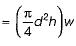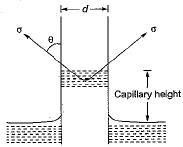Vertical component of surface tension force
= σ cosθ x circumference
= σ cosθ x πd = πdσcosθ
In equilibrium, the downward weight of the liquid column h the balanced by the vertical component of the force of surface tension.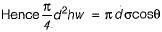Height of capillarity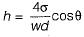QUESTION: 9

The approximate value of θ (angle of contact) for mercury is

Solution: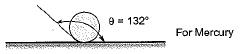QUESTION: 10

What is the dimensions of kinematic viscosity of a fluid?

Solution:

v = m2/s = L2T-1

QUESTION: 11

The property by virtue of which a liquid opposes relative motion between its different layers is called

Solution:

From the definition of viscosity, viscosity is the resistance offered by the one layer of fluid on another layer and opposes relative motion between.

QUESTION: 12

The Bulk modulus of elasticity of a fluid is defined a

Solution:

Bulk modulus of elasticity (k)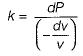Also,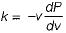It represents change in volume with pressure.

QUESTION: 13

In the phenomenon of cavitation, the characteristic fluid property involved is

Solution:

In any flow system, if the pressure at any point in the liquid approaches the vapour pressure, vaporization of liquid starts, resulting in the pockets of dissolved gasses and vapours. The bubbles of vapour thus formed are carried by the flowing liquid into a region of high pressure where they collapse, giving rise to high impact pressure. This phenomenon is known as cavitation.

QUESTION: 14

The difference of pressure between the inside and outside the soap bubble is

Solution:

A spherical soap bubble has surfaces in contact with air, one inside and the other outside, each one of which contributes the same amount of tensile force due to surface tension. As such on a hemispherical section of a soap bubble of radius r the tensile force due to surface tension in equal to 2σ(2πr). However, the pressure force acting on the hemispherical section of the soap bubble is same as in the case of a droplet and it is equal to ρ(πr2). Thus equating these two forces for equilibrium, we have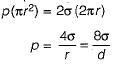QUESTION: 15

The difference of pressure between the inside and outside the liquid jet

Solution:

Consider a jet of liquid of radius r, length l and having internal pressure intensity p is excess of the outside presure intensity. If the jet is cut into two halves, then the forces acting on one half will be those due to pressure intensity p on the projected area (2rl) and the tensile force due to surface tension a acting along the two sides (2l).
These two forces will be equal and opposite for equilibrium and hence we have
ρ(2rl) = σ(2l)
or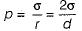QUESTION: 16

The difference of pressure between the inside and outside the liquid drop is

Solution:

Consider a spherical droplet of radius r having internal pressure intensity ρ in excess of the outside pressure intensity. If the droplet is cut into two halves, then the forces acting on one half will be those due to pressure intensity p on the projected area (πr2) and the tensile force due to surface tension a acting around the circumference (2πr). These two forces will be equal and opposite for equilibrium and hence we have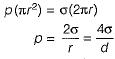QUESTION: 17

Match List-I (Properties of fluids) with List-ll (Definition) and select the correct answer using the codes given below the lists:
List-I
A. Ideal fluid
B. Newton ion fluid
C. μ/ρ
D. Mercury in glass
List-ll
1. Viscosity does not vary with rate of deformation
2. Fluid of zero viscosity
3. Dynamic viscosity
4. Capillary depression
5. Kinematic viscosity
6. Capillary rise
Codes:
A  B  C D
(a) 1  2  4  6
(b) 1  2  3  4
(c) 2  1  3  6
(d) 2  1  5  4

Solution:

Ideal fluid is non-viscous and incompressible
For Newton ion fluid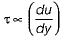Kinematic viscosity v = μ/ρ
Mercury in glass - Capillary depression

QUESTION: 18

The capillary rise at 20°C in clean glass tube of 1 mm diameter containing water is

Solution: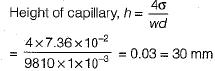QUESTION: 19

If the surface tension of water air interface is 0.073 N/m, the gauge pressure inside a rain drop of 1 mm diameter will be

Solution: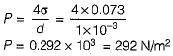QUESTION: 20

Consider a soap film bubble of diameter D. If the external pressure is P0 and the surface tension of the soap film is σ, the expression for the pressure inside the bubble is

Solution: